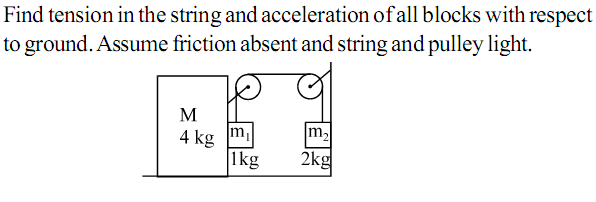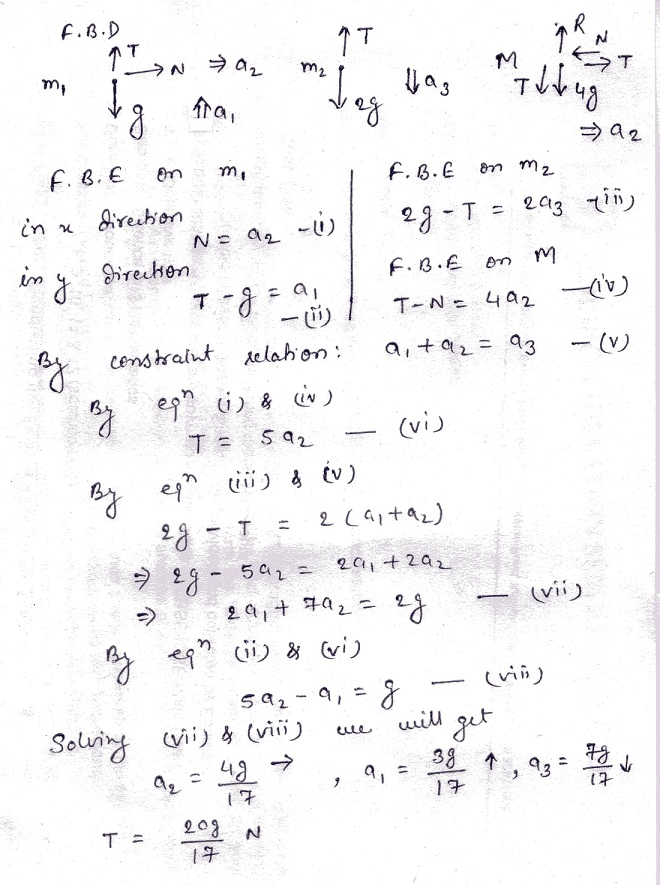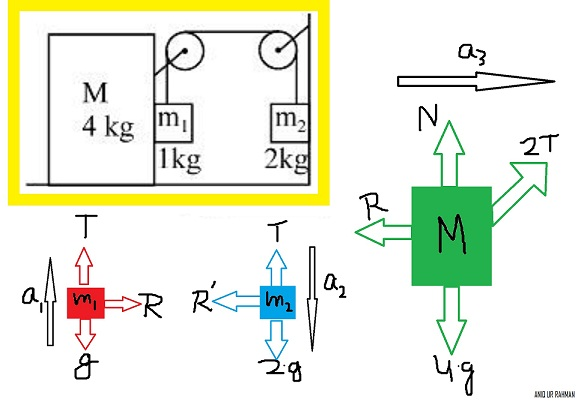# Laws of Motion-21•Akash Anand ·•Niraj kumar Jha ·

acc. of m2 g/3 downward.
acc. of m1 g/3 upward.
acc. of M 2g/3 rightward.
tension in the joining m1&m2 is 4g/3

Sourish Ghosh ·

m2 = g/3 down
m1 = g/3 up and 4g/15 right
M = 4g/15 right
T = 4g/3

Akash Anand ·

All of you have got the wrong answer. This is a very conceptual question of Constraint Relation. All I can give you is a hint for now. Acceleration of 2 kg block and 1 kg block in vertical direction will not be the same (in magnitude). If we take acceleration of 1 kg block as a1 (in upward direction) and acc. of 2 kg as a2 (downward) and acc. of 4 kg as a3 in rightward then constraint relation will be: a2=a1+a3 (Think why??)

Swarna Kamal Dhyawala ·

a1=107 m/s2 (for block of mass 1kg)
a2=307 m/s2 (for block of mass 2kg)
a3=207 m/s2 (for block of mass 4kg)
T=807N

•Aniq Ur Rahman ·

Are the F.B.Ds correct.
I'm having difficulty in finding the constraint relation .

•Aniq Ur Rahman ·Are the F.B.Ds correct?
I'm having difficulty in finding the constraint relation .